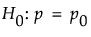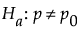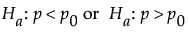Publication date: 07/30/2020

# One Sample Proportion Calculator

Use the One Sample Proportion calculator to evaluate sample size for a hypothesis test about one proportion. Sample size and power are associated with the following hypothesis test:versus the two-sided alternative:or versus a one-sided alternative:where p is the population proportion and p0 is the null proportion.

The One Proportion Calculator also provides the actual test size. This is the actual Type I error rate for the specified assumptions. Since the binomial distribution is discrete, the actual test size can differ significantly from the stated Alpha level for small samples or proportions near 0 or 1. To guarantee an alpha level equal to or greater than your stated level, use the Exact Clopper-Pearson method.